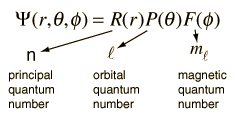#### Thank you for registering.

One of our academic counsellors will contact you within 1 working day.

Click to Chat

1800-5470-145

+91 7353221155

CART 0

• 0
MY CART (5)

Use Coupon: CART20 and get 20% off on all online Study Material

ITEM
DETAILS
MRP
DISCOUNT
FINAL PRICE
Total Price: Rs.

There are no items in this cart.
Continue Shopping

# how solutions of Schrodinger's equation contribute towards electron density curve of hydrogen atom?

11 years ago

Dear anurag,

The solution of the Schrodinger equation for the hydrogen atom is a formidable mathematical problem, but is of such fundamental importance that it will be treated in outline here. The solution is managed by separating the variables so that the wavefunction is represented by the product:The separation leads to three equations for the three spatial variables, and their solutions give rise to three quantum numbers associated with the hydrogen energy levels.

Please feel free to post as many doubts on our discussion forum as you can.

All the best.

### General quantum system

For a general quantum system:$i\hbar\frac{\partial}{\partial t} \Psi = \hat H \Psi$

where

• Ψ is the wave function; the probability amplitude for different configurations of the system at different times,
•$i \hbar \frac {\partial}{\partial t}$ is the energy operator (i is the imaginary unit and$\hbar$ is the reduced Planck constant),
•$\hat H$ is the Hamiltonian operator.

### Single particle in a potential

For a single particle with potential energy V, the Schrödinger equation takes the form:$i\hbar\frac{\partial}{\partial t} \Psi(\mathbf{r},\,t) = -\frac{\hbar^2}{2m}\nabla^2\Psi(\mathbf{r},\,t) + V(\mathbf{r})\Psi(\mathbf{r},\,t)$

where

•$-\frac{\hbar^2}{2m}\nabla^2$ is the kinetic energy operator, where m is the mass of the particle.
•$\nabla^2$ is the Laplace operator. In three dimensions, the Laplace operator is$\frac{\partial^2}{{\partial x}^2} + \frac{\partial^2}{{\partial y}^2} + \frac{\partial^2}{{\partial z}^2}$, where x, y, and z are the Cartesian coordinates of space.
•$V\left(\mathbf{r}\right)$ is the time-independent potential energy at the position r.
•$\Psi(\mathbf{r},\,t)$ is the probability amplitude for the particle to be found at position r at time
11 years ago

schrodinger equation is a differential equation written in terms of electron wave function. So, the equation is a store house of information  about the revolving electron. As squre of amplitude speaks about the intensity of a wave, square of si gives probability of finding the electron.

4(pi) r2 gives area of sphere, 4(pi) r2 dr gives a volume segment.

4(pi) r2 dr (si) square gives radial probability distribution in a volume segment with out the consideration of any direction, that is nothing but electron cloud density,